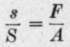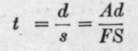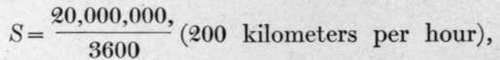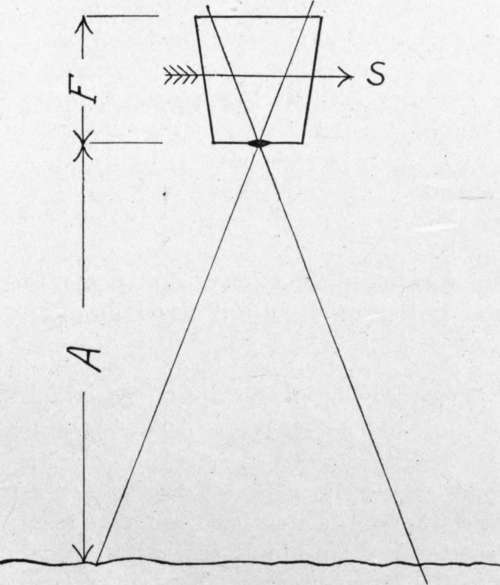A definite limitation to the length of exposure in airplane cameras is set by the motion of the plane. If we represent the speed of the plane by S, the altitude of the plane by A, and the focal length of the lens by F, we obtain at once from the diagram (Fig. 19), that s, the rate of movement of the image on the plate, is given by the relation,.

If we call the permissible movement d, then the permissible exposure time, t, is given by the relation.

As a representative numerical case, expressing all quantities in centimeters and in centimeters per second, let F = 50,and A = 300,000, then.

If we take for the permissible undetectable movement, .01 centimeter, which is, as has been shown, a reasonable figure for lens defining power, we have, then, that the longest permissible exposure is .011 secondin round numbers, one-hundredth.

In flying with a slow plane, or in flying against the wind, the exposure can sometimes be increased to as much as double this length. Diminishing F would similarly extend the allowable exposure, but the ratio of F to A approximates has, of course, been derived from war-time experience. Probably much of the prospective peace-time mapping work will impose substantially easier requirements as to definition and will thus allow longer exposures.Fig. 19. - Relative motion of plane and photographic image.

For low oblique views the longest exposure is much less. Taking 45 degrees as a representative angle for the foreground, and 500 meters as a representative height, the value of t becomes -^-q to a constant in actual practice; in other words, a certain resolution and size of image have been found desirable. If flying is forced higher, a longer focus lens is used; if lower flying is possible, a lens of shorter focus. This relationship: m>s.

These figures will illustrate two important points: they show how severe is the limitation as to exposure, with the consequent heavy demand on lens and sensitive material speed; and they show how important it is to secure a shutter with the maximum light-giving power for a specified length of exposure. This leads to a study of the characteristics as to efficiency of the two common types of shutter, namely, shutters at or between the lens, and focal-plane shutters.